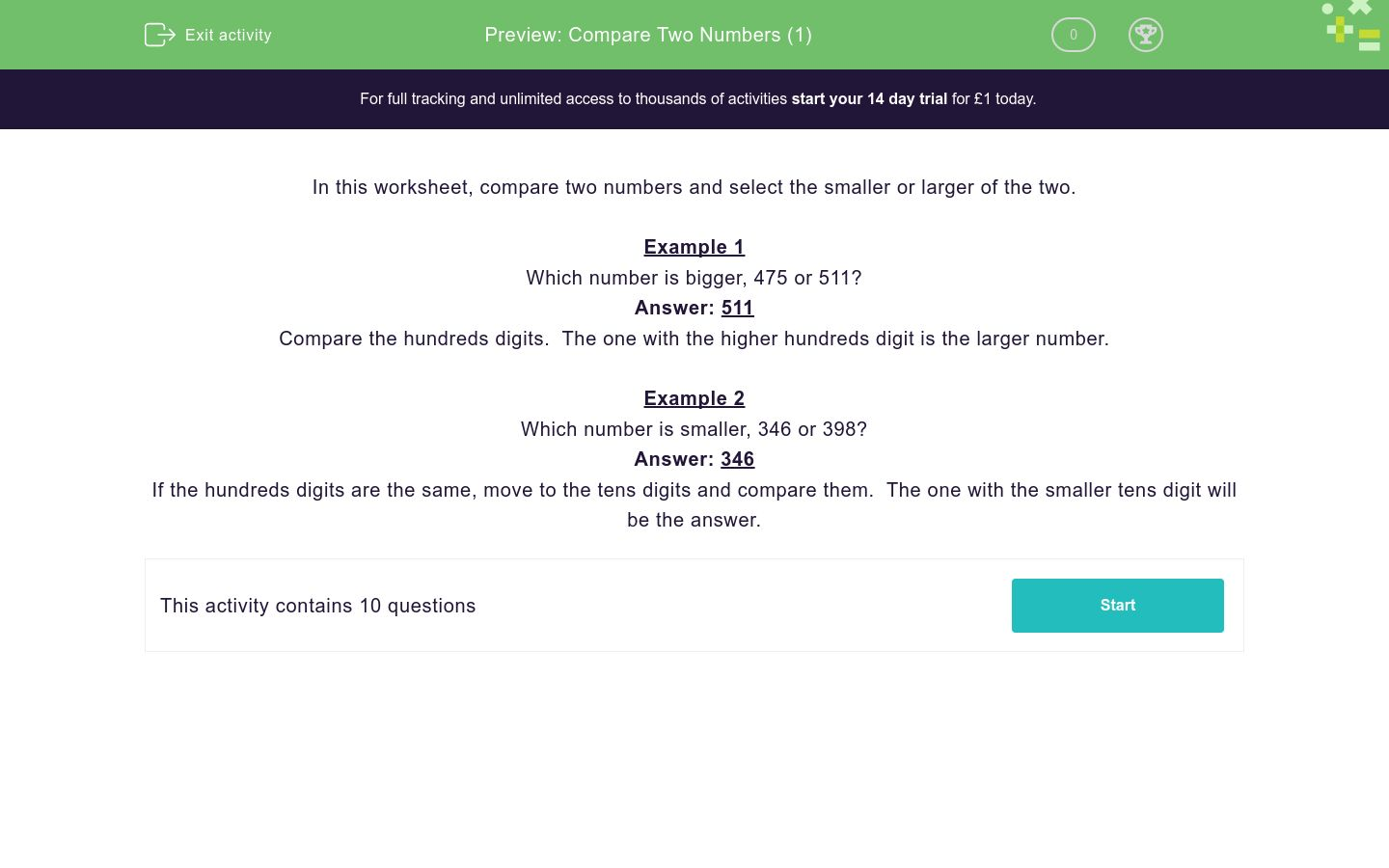# Compare Two Numbers (1)

In this worksheet, students compare numbers and pick out the larger or smaller of the two numbers.Key stage:  KS 2

Curriculum topic:   Number: Number and Place Value

Curriculum subtopic:   Order/Compare Numbers to 1000

Difficulty level:### QUESTION 1 of 10

In this worksheet, compare two numbers and select the smaller or larger of the two.

Example 1

Which number is bigger, 475 or 511?

Compare the hundreds digits.  The one with the higher hundreds digit is the larger number.

Example 2

Which number is smaller, 346 or 398?

If the hundreds digits are the same, move to the tens digits and compare them.  The one with the smaller tens digit will be the answer.

Which number is smaller, 612 or 502?

612

502

Which number is larger, 293 or 371?

293

371

Which number is smaller, 331 or 377?

331

377

Which number is larger, 570 or 380?

570

380

Which number is smaller, 867 or 237?

237

867

Which number is greater, 147 or 780?

147

780

Which number is larger, 153 or 980?

153

980

Which number is larger, 484 or 126?

484

126

Which number is smaller, 540 or 595?

540

595

Which number is higher, 426 or 336?

426

336

• Question 1

Which number is smaller, 612 or 502?

502
EDDIE SAYS
Compare the numbers in the hundreds place. 500 is less than 600, so 502 is the smaller number.
• Question 2

Which number is larger, 293 or 371?

371
EDDIE SAYS
Compare the numbers in the hundreds place. 300 is greater than 200, so 371 is the greater number.
• Question 3

Which number is smaller, 331 or 377?

331
EDDIE SAYS
Compare the numbers in the hundreds place. They are both 300. Now compare the numbers in the tens place. 30 is less than 70, so 331 is the smaller number.
• Question 4

Which number is larger, 570 or 380?

570
EDDIE SAYS
Compare the numbers in the hundreds place. 500 is greater than 300, so 570 is the greater number.
• Question 5

Which number is smaller, 867 or 237?

237
EDDIE SAYS
Compare the numbers in the hundreds place. 200 is greater than 800, so 237 is the smaller number.
• Question 6

Which number is greater, 147 or 780?

780
EDDIE SAYS
Compare the numbers in the hundreds place. 700 is greater than 100, so 780 is the greater number.
• Question 7

Which number is larger, 153 or 980?

980
EDDIE SAYS
Compare the numbers in the hundreds place. 900 is greater than 100, so 980 is the greater number.
• Question 8

Which number is larger, 484 or 126?

484
EDDIE SAYS
Compare the numbers in the hundreds place. 400 is greater than 100, so 484 is the greater number.
• Question 9

Which number is smaller, 540 or 595?

540
EDDIE SAYS
Compare the numbers in the hundreds place. They are both 500. Now compare the numbers in the tens place. 40 is less than 90, so 540 is the smaller number.
• Question 10

Which number is higher, 426 or 336?

426
EDDIE SAYS
Compare the numbers in the hundreds place. 400 is greater than 300, so 426 is the greater number.
---- OR ----

Sign up for a £1 trial so you can track and measure your child's progress on this activity.

### What is EdPlace?

We're your National Curriculum aligned online education content provider helping each child succeed in English, maths and science from year 1 to GCSE. With an EdPlace account you’ll be able to track and measure progress, helping each child achieve their best. We build confidence and attainment by personalising each child’s learning at a level that suits them.

Get started